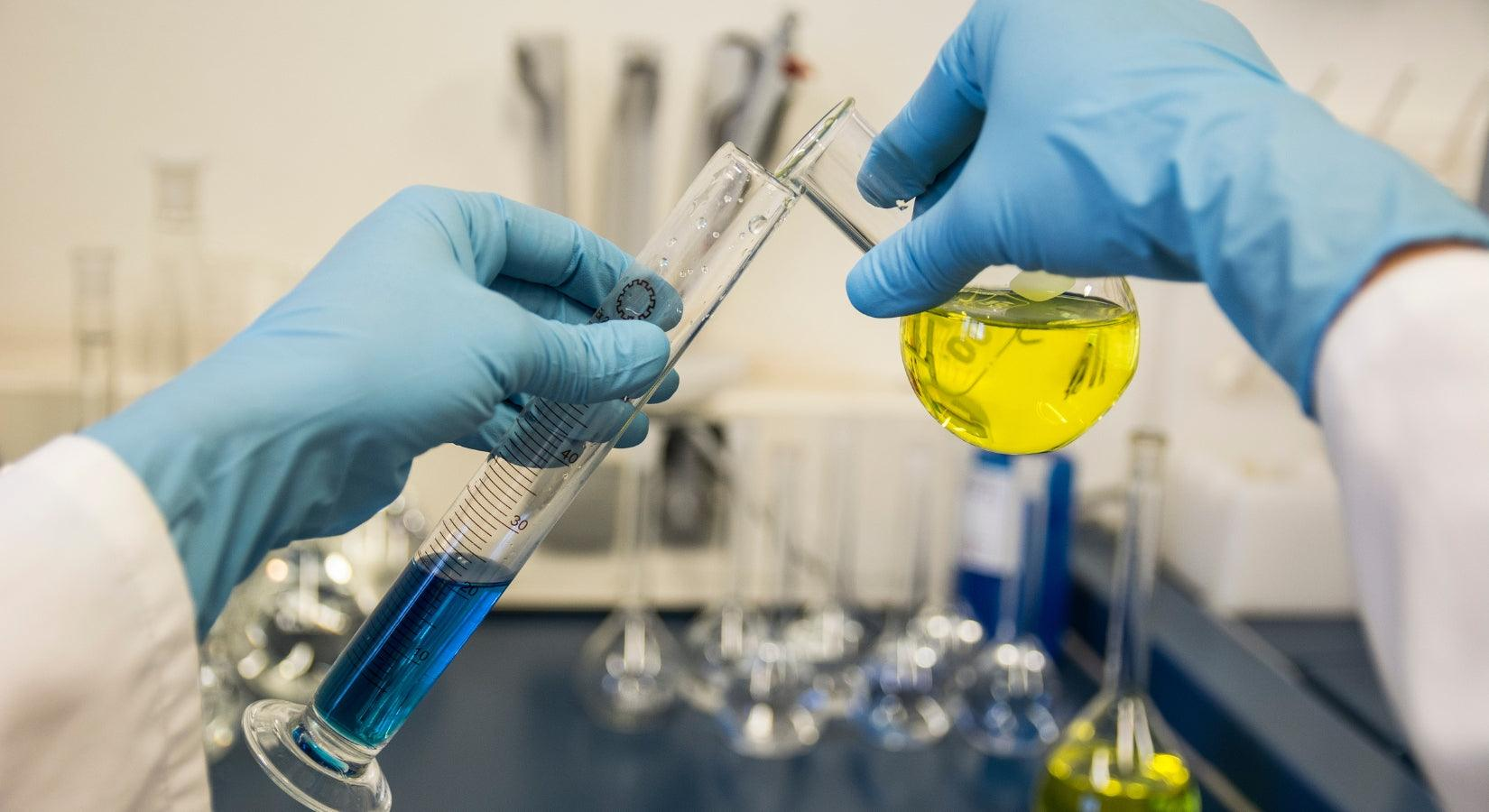HIRANUMA APPLICATION DATA Automatic Titrator Data No. K8 Feb. 10,2021
 Organic acid Fractional determination of ascorbic acid and sodium ascorbate

## 1. Abstract

Ascorbic acid has properties as an acid and a strong reducing agent. On the other hand, sodium ascorbate doesn’t have a function as an acid but works as a reducing agent as well as ascorbic acid. The quantitative determination method for ascorbic acid is prescribed in JIS K 9502 and Japanese pharmacopeia. There are two determination methods for ascorbic acid; neutralization titration and iodine titration.

The fractional determination method for ascorbic acid and sodium ascorbate is introduced in this report. First, ascorbic acid is determined by the neutralization titration with sodium hydroxide standard solution (formula (1)). After that, the total amount of ascorbic acid (ascorbic acid and sodium ascorbate) is measured by the redox titration with iodine standard solution (formula (2) and (3)). The sodium ascorbate is determined by the subtraction of the ascorbic acid from the total amount of ascorbic acid.

 (I) Reaction formula for neutralization titration C₆H₈O₆ ＋ NaOH → C₆H₇NaO₆ ＋ H₂O ・・・(1) (II) Reaction formulae for redox titration C₆H₈O₆ ＋ I₂ → C₆H₆O₆ ＋ 2HI ・・・(2) C₆H₇NaO₆ ＋ I₂ → C₆H₅NaO₆ ＋ 2HI ・・・(3)

## 2. Configuration of instruments and Reagents

 (1) Configuration Main unit : Automatic Titrator COM series Option : One buret and one buret head Electrode : Glass reference electrode　GR-501BZ　Connect to IE-1.Platinum electrode　PT-301　Connect to IE-2. (2) Reagents Titrant : 0.1 mol/L Sodium hydroxide standard solution0.05 mol/L Iodine standard solution Additive : 2 % Metaphosphoric acid solution

## 3. Measurement procedure

 (1) Measurement for ascorbic acid (i) Take approx 0.4 g of sample into a 100 mL beaker and weigh it accurately. (ii) Add approx 40 mL of DI water. (iii) Immerse the electrodes to start titration with 0.1 mol/L sodium hydroxide standard solution.

 (2) Measurement for sodium ascorbate (i) Take approx 0.4 g of sample into a 100 mL beaker and weigh it accurately. (ii) Add 50 mL of 2 % metaphosphoric acid solution. (iii) Immerse the electrodes to start titration with 0.05 mol/L iodine standard solution with using optional buret and buret head.

## 4. Measurement conditions and results

#### Examples of titration conditions

(1) Measurement for ascorbic acid

 Cndt No. 1 Method Auto Buret No. 1 Amp No. 1 D. Unit pH S-Timer 5 sec C.P. mL 0 mL T Timer 0 sec D.P. mL 0 mL End Sens 200 Over mL 0.2 mL Max Vol. 20 mL Constant No. 1 Size 0.4036 g Blank 0 mL Molarity 0.1 mol/L Factor 1.004 K 176.13 L 0 Unit mg/g Formula (D-B)*K*F*M/S Decimal Places 4 Auto input Param. None Mode No. 4 Pre Int 0 sec Del K 9 Del Sens 0 mV Int Time 3 sec Int Sens 3 mV Brt Speed 2 Pulse 40

(2) Measurement for sodium ascorbate

 Cndt No. 2 Method Auto Buret No. 2 Amp No. 2 D. Unit mV S-Timer 30 sec C.P. mL 7 mL T Timer 30 sec D.P. mL 0.5 mL End Sens 500 Over mL 0.2 mL Max Vol. 20 mL Constant No. 2 Size 0.4042 mL Blank 0 mL Molarity 0.05 mol/L Factor 1.001 K 176.13 L 0 Unit mg/g Formula (D-B)*K*F*M/S Decimal Places 4 Auto input Param. None Mode No. 20 Pre Int 0 sec Del K 2 Del Sens 0 mV Int Time 3 sec Int Sens 3 mV Brt Speed 2 Pulse 40

 Cndt No. 3 Method Calc Constant No. 3 Size 0.4042 g Blank 0 mL Molarity 0 mol/L Factor 0 K 116.92 *1 L 1.125 *2 Unit mg/g Formula (CA-K)*L Decimal Places 4 Auto input Param. None

*1 K (coefficient 1): Result of ascorbic acid by neutralization titration
*2 L (coefficient 2): Coefficient to convert ascorbic acid to sodium ascorbate (C₆H₇NaO₆ (198.11) / C₆H₈O₆ (176.13))

#### Measurement results

Measurement results of ascorbic acid

Meas.
No.
Size
(g)
Titrant
volume(mL)
Ascorbic acid
(mg/g)
Statistic calculation
1 0.4036 2.671 117.028 Avg. 116.92 mg/g
2 0.4021 2.659 116.931 SD 0.12 mg/g
3 0.4055 2.678 116.796 RSD 0.10 %

#### Examples of titration curves#### Measurement results

Measurement results of sodium ascorbate

Meas.
No.
Size
(g)
Titrant
volume(mL)
Total ascorbic acid
(mg/g)
Sodium ascorbate
(mg/g)
Statistic calculation
1 0.4042 9.858 215.001 110.341 Avg. 110.70 mg/g
2 0.4056 9.922 215.671 111.095 SD 0.38 mg/g
3 0.4064 9.925 215.275 110.649 RSD 0.34 %

#### Examples of titration curves## 5. Note

Another method for successive titration

The iodine titration method is used for the determination of ascorbic acid in this report, but there is an indophenol method as another determination method for ascorbic acid; the color change of indophenol from blue to red under metaphosphoric acid is detected as the end point of titration in this method. The relatively-high selectivity on the determination of ascorbic acid in fruit juice etc. compared with the iodine titration method is the character of the indophenol method.

Keywords： Fractional determination of ascorbic acid and sodium ascorbate, Neutralization titration, Redox titration, Iodine titration

*Some measurement would not be possible depending on optional configuration of system.

Featured Instruments

View all